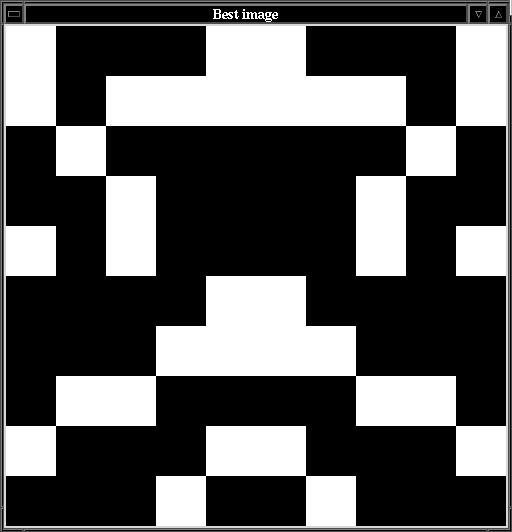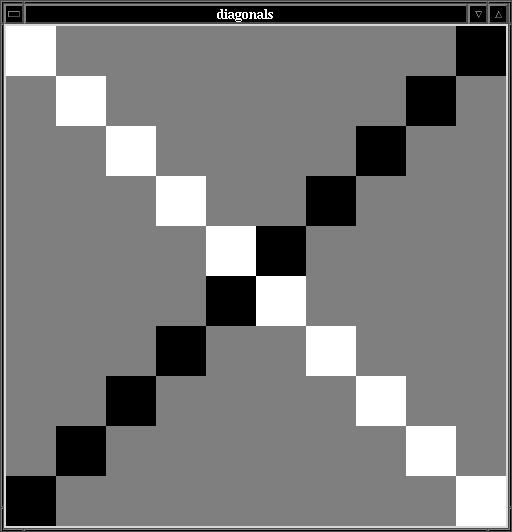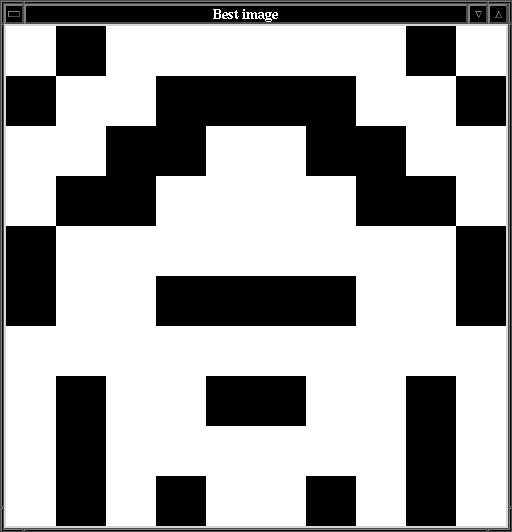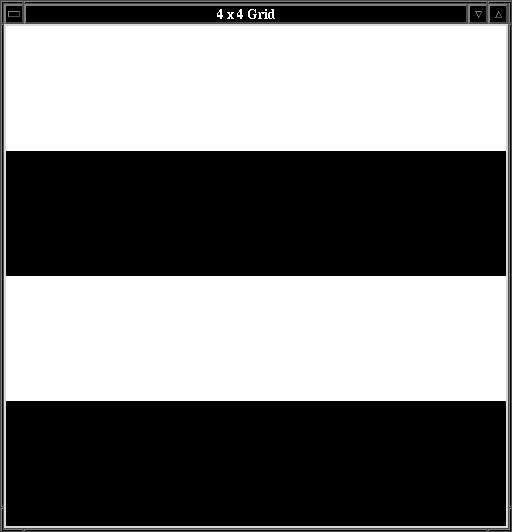# CS63 Lab 4: Genetic Algorithm

Due by 11:59pm Thursday, Nov. 3

A skeleton version of the programs will appear when you run update63 in a terminal window.

Introduction

A genetic algorithm is an abstract simulation of some of the key ideas from biological evolution. A population of candidate solutions, where each candidate solution is represented as a series of 1's and 0's, is randomly generated. Then evolution proceeds as a sequence of generations. Each generation, the fitness of every candidate solution in the population is determined. Next, parents are selected from the population based on their fitness. With some probability, a crossover operation is done on these parents. Crossover is a simple model of sexual reproduction that recombines the genetic material of two parents to produce two children. Finally, with some low probability, mutation may occur on the children's' genetic material. In this way, a new population is produced on each generation. Over many generations, the average fitness of the population tends to increase and more highly fit candidate solutions are created.

In this lab you will be given a skeleton definition of a GeneticAlgorithm class that you will complete. Then you will test the completed genetic algorithm on a simple problem of finding candidate solutions that maximize the sum of their values.

Once you are convinced that the genetic algorithm is working properly, you will then apply it to the more interesting problem of evolving symmetrical grid-based images. An example is shown below.The GeneticAlgorithm class

Open the ga.py file that was copied into your cs63/labs/4 directory when you did an update63. Read through the methods that you will need to implement. Recall that there are three main operators used in a genetic algorithm: selection, crossover, and mutation. Below is a review of how they should function.

• The selection method takes no parameters and stochastically chooses a candidate solution from the current population based on its fitness. The probability of choosing a particular candidate solution is the ratio of its fitness to the total fitness of the entire population. Here is a method for achieving this:
```compute totalFitness of population
spin = random number between 1 and the totalFitness
partialFitness = 0
for each individual in the population:
partialFitness += fitness(individual)
if partialFitness >= spin:
return individual
```
• The crossover method takes two parents and a probability as input. The parameter probCross represents the probability that crossover will occur for those two parents. When crossover doesn't occur, copies of the two parents should be returned. Recall that you can get a copy of a python list ls by creating a slice of the entire list ls[:]. When crossover does occur, a random crossover point between 1 and the length of the parent-1 should be determined. For example, if the parent was 16 long, then the crossover point should be randomly chosen to be between 1 and 15. The first child should be created by taking parent1's values starting from the beginning and continuing up to, but not including the crossover point. The remainder of the first child should be created by taking parent2's values starting from the crossover point and continuing to the end. The second child should be created using the opposite portions of the two parents. An example is shown below.
```
0  1  2  3  4  5  6  7
parent1: [1, 1, 1, 0, 0, 0, 0, 0]
parent2: [0, 1, 0, 1, 0, 1, 0, 1]
^
crossPoint:       3

child1:  [1, 1, 1, 1, 0, 1, 0, 1]
child2:  [0, 1, 0, 0, 0, 0, 0, 0]
```
Test that your crossover method is working properly by printing out the parents, the selected crossover point, and the resulting children.
• The mutation method takes a child and a probability as input, and for each position in the child it uses the probability to determine whether that particular position should be modified. When making a mutation, if the current value is a 0 then it is changed to a 1, and if the current value is a 1 is is changed to a 0. An example is shown below.
```child before mutation:  [1, 1, 1, 1, 0, 1, 0, 1]
child after  mutation:  [1, 0, 1, 1, 0, 1, 0, 1]
```
Because the child is represented as a list, any change you make will be permanent, so it does not need to be returned from the method.

Test that your mutation method is working properly by printing out the incoming child and the child after mutation is completed. Remember that it is possible for none of the child to be mutated and it is also possible for multiple positions on the child to be mutated.

Once you have implemented all of the class methods, test whether you can evolve a solution of all 1's using the class called SumGA provided at the bottom of the ga.py file.

If you cannot consistently evolve a solution of all 1's, then there may be a problem with your genetic operators or with your methods that control the evolutionary process.

The Grid class

Before you begin evolving images, you'll need to understand how to use the Grid class to draw two-dimensional, grid-based images.

To construct a Grid object, you'll need to pass the desired number of columns and rows as parameters. The origin (0,0) is located in the bottom left corner. Once you have a grid object you can set the color of particular cells as well as set the title. For example, try the following code to produce the picture shown below.

```from Grid import *
g = Grid(10,10)
g.setTitle("diagonals")

for i in range(g.getWidth()):
g.setBlack(i, i)

for i in range(g.getWidth()):
g.setWhite(i, g.getWidth()-i-1)

g.update()
g.done()
```

Notice that you must call the update method to actually make the window update its color values.Once you are comfortable using the Grid class and its methods, you can move on to the next section.

The GAImage class

Using the SumGA class given in the ga.py file as a model, complete the implementation of the ImageGA class given in the imageGA.py file. Your goal is to create a fitness function that can evolve images that are symmetrical across the vertical axis. Another example is shown below.1. Create a constructor for the GAImage class that takes the columns, rows, and population size as parameters. It should create a blank Grid object and save it as a class variable.
2. Create a showImage method that takes a candidate solution and draws it in the Grid object. You should interpret 1's as white cells and 0's as black cells. For example, the following candidate solution of length 16,
```[0, 0, 0, 0, 1, 1, 1, 1, 0, 0, 0, 0, 1, 1, 1, 1]
```
would represent this 4x4 image, where the the origin is in the lower-left corner:3. Create a fitness method that takes a candidate solution and returns a score that represents how symmetrical the associated image would be. You can count the number of cells in symmetrical positions across the vertical axis which have the same value. As in the simpler SumGA example, you should base the fitness on a power of two of the count so as to speed up the process of evolution.
4. Create an isDone method. It should use the showImage method to display the best member of the current generation so that you can watch the progress of the evolution. It should also test if the fitness of the best member of the current generation is equal to the optimal fitness for vertical symmetry. If so, it should return True, otherwise it should return False.
5. Create a main program to test your class. Be sure that your main program includes a line that calls the done method of the grid associated with your genetic algorithm. This will wait for a mouse click before ending the program and will allow you to view the best image found.

When testing on a 10 by 10 grid, you should be able to evolve symmetrical images with the following parameters:
• population size 50
• generations 100
• probability of crossover 0.6
• probability of mutation 0.01 (this is higher than is typical)

Optional extensions

Once you have successfully created a GA that can evolve images with vertical symmetry, feel free to try other ideas, such as:

• Evolve images with horizontal symmetry.
• Evolve images to fit some other pattern of your choice.
• Evolve images with more than two colors. The Grid class includes the method setColor that allows you to set a cell to any combination of red, green, and blue values. Read help(Grid) to find out more. In order to use the GA to evolve images with more than two colors, you will need to interpret the candidate solutions differently. Rather than having one bit represent the color of one cell, you would need to use several bits to represent the color of one cell.
• Evolve solutions to something completely different. As long as you can represent your task as a series of 1's and 0's and can define a fitness function that can return a relative measure of success, you can apply the genetic algorithm to that task.

In comments, be sure to give a clear explanation of your fitness function and your goal for the evolutionary process.

Submit

Once you are satisfied with your program, hand it in by typing handin63 in a terminal window.